Contraction

Contraction is one of the most important operations in tensor analysis. Lets illustrate the operations using the two tensors A,Y below.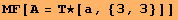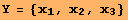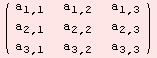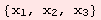The matrix-vector product can be interpreted as an outer product followed by 2,3-contraction. The command for contraction is ΤC.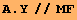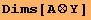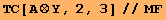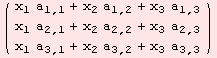Lets move to a more complicated example. Consider the tensor X below.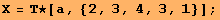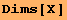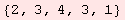The only contraction we can perform on X is on dimensions 2 and 4, refered to 2,4-contraction. Have a look at the indicees, to understand how the computation is done. The remaining dimensions of the contracted tensor are 2,4,1.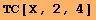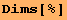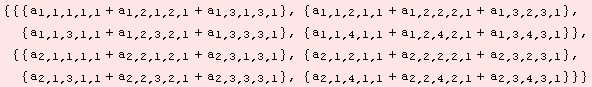We get an error for any other combination such as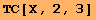The 1,2-contraction of a square matrix X is simply the trace of the matrix X: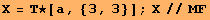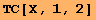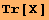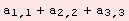If the dimensions to be contracted on are not specified, then 1,2-contraction is performed.Created by Mathematica  (September 30, 2006)